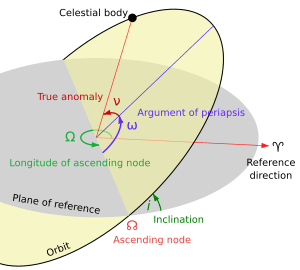# Orbital plane (astronomy) facts for kids

Kids Encyclopedia FactsAn orbital plane as viewed relative to a plane of reference

An orbital plane is the geometric plane of an orbit. It is described by its difference from a reference plane.

The reference plane for the Solar System is usually the Earth's orbital plane. This defines the ecliptic, the path on the celestial sphere that the Sun appears to follow over a year.

For example, suppose a planet is orbiting around a star in an elliptical orbit. The area within the path is the 'orbital plane' of that planet. The plane of the Earth's orbit around the Sun is called the ecliptic plane.

Sometimes the invariable plane is used instead.Orbital plane (astronomy) Facts for Kids. Kiddle Encyclopedia.